# Add a Monte Carlo simulation

Add a Monte Carlo simulation to explore the behavior of a complex system or process.

## Define the model and run the simulation

To run a simulation, you must have the information necessary to specify the model:
• The distribution and parameters for each input. For more information, see "Choose a distribution" in this topic.
• The equations that describe your system or process. These may be known equations, or they may be based on a model that you created from a designed experiment (DOE) or regression analysis in Minitab. For more information, see "Import Models" in this topic.

After you collect this information, you are ready to run a simulation.

1. Choose Insert > Monte Carlo Simulation.
2. In the Roadmap, rename the simulation so it's meaningful to you.
3. (Optional) Click Import Models from Minitab. You can choose a Minitab project that you added as a related document or one that you saved to your computer or a network. For more information, see "Import Models" in this topic.
4. For each input:
• Enter a name that describes the input. If you imported a model from Minitab, you can change the name of the imported input.
• Choose a distribution, and then enter its parameters.###### Tip

If you're not sure which distribution to choose and you have historical data from the system, choose Use data to decide. Then, browse to the CSV file that contains data. Be sure that the data represents expected future performance. Workspace automatically finds the best-fitting distribution. For more information, see "Choose a distribution" in this topic.

5. For each output:
• Enter a name that describes the output. If you imported a model from Minitab, you can change the name of the imported output variable.
• If you didn't import a model from Minitab, build the equation using the equation editor.6. (Optional) Enter a lower specification limit, an upper specification limit, or both. To get process performance metrics, you must enter at least one specification limit. For more information, see "Calculating Cpk and Ppk in simulations" in this topic.
7. Use the diagram to verify your model.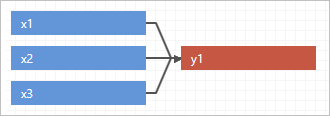8. On the Simulation menu, enter the number of iterations. The number of iterations should adequately cover the range of possible input and output values, and provide accurate results. The maximum number is 1,000,000. However, the default of 50,000 is enough for most models.9. Choose Simulation > Simulate.

## Create groups

When you have a complex or a large simulation, you can create groups to define the model by function. For example, you might want to describe different actions or various parts' behavior within the simulation. With groups, you can categorize inputs and outputs to help you manage and organize your simulation.

1. After you insert a simulation, choose Simulation > Create Group. A new tab appears above the x variables.2. To rename this group, double-click the tab and enter a name.
3. To add another group, click the plus sign icon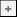beside the new group. If you accidentally close a group, press Ctrl+Z to undo the action.
4. Enter new inputs and outputs for each group. If an input or an output is used in more than one group, you only need to enter it once.
5. Build the output equation for each group. Inputs and outputs from all groups are available in the equation editor.
6. Use the diagram to verify the model, by model or function, for each group or for all groups.
To view all groups, click All Groups above the distribution previews.The following example shows all groups by model.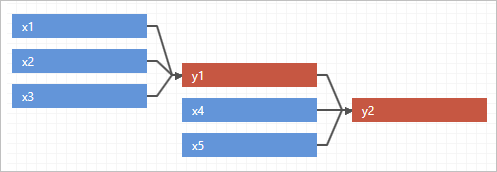The following example shows all groups by function.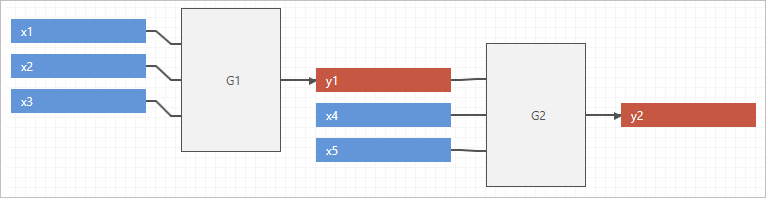## Choose a distribution

One of the most challenging tasks may be selecting the appropriate distribution and parameters needed for each input variable. Although each application is different, you can use the following guidelines:
• If historical data is available and represents expected future performance, you can use Workspace's Use data to decide feature. Workspace automatically finds the best-fitting distribution.
• If historical data is not available and you cannot collect data on the past performance of the system, consider these alternatives:
• Talk to others in your organization that may have insight into the system.
• Consult the literature. Be sure to consider the source and look for industry-specific examples that match your application.

## Import models from Minitab

Workspace can import any number of models from Minitab 19 or later. When you import models, Workspace imports the following:
• X variables (inputs), which can include continuous factors, categorical factors, and covariates
• Y variables (outputs)
• The Y equation, along with the inputs' coefficients (in uncoded units) and the constant term

If a model contains categorical factors, you can select the factor levels to include in the equation.

###### Note

To compare the simulation results for the same y variable using different factor levels, select the y variable and the factor level to include in the equation, then import them. Repeat this process until you have selected all the factor levels to compare.

## Calculating Cpk and Ppk in simulations

In Monte Carlo simulations, it is typical for simulated responses to violate the assumption of normality. Therefore, Workspace uses a nonparametric method to calculate capability in the simulation tool because it works for both normal and nonnormal data. The nonparametric method calculates the spread of the output distribution using the observed 0.135 and 99.865 percentiles of the simulated data, which is analogous to +/-3 sigma in a normal distribution.

Because there are no subgroups and no concept of long term and short term variation in the simulation context, Cpk and Ppk values are equivalent in the Workspace Monte Carlo simulation. You can choose which label to display in the simulation results. Choose File > Options and select the label you prefer.

Based on the spread in the data and the specification limits you set in the model, Workspace calculates ppl and ppu to find the corresponding Ppk. As with typical capability calculations, Workspace takes the distances between the process "center" and each spec and divides by the spread of the distribution to get ppl and ppu. The smallest value is chosen to represent the capability.

Min (ppl, ppu) where:
• ppl = (median-lsl)/(median - 0.135th percentile)
• ppu = (usl - median)/(99.865th percentile - median)

## Examine the results

Workspace displays the results of the simulation, how your results compare to generally accepted values, and guidance for next steps.

1. View the results in the workspace. The business question for the construction projects is "What percent of projects will take more than 30 business days?". Based on these results, you can expect around 15% of the projects to extend past 30 days.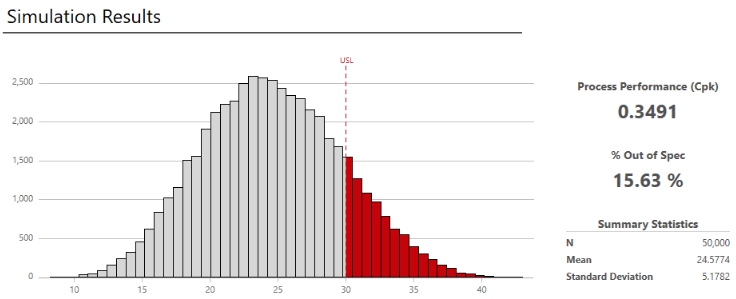###### Note

Each time you repeat the simulation, the results will vary because the simulation is based on randomly selected values for the inputs.

2. You can do any of the following tasks:
• To view DPMO (Defects Per Million Opportunities), observed performance, summary statistics, percentiles, and model assumptions, click More Results.
• To view information about next steps, hold the pointer on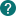.
• To add or change the specification limits, choose Simulation > Specification Limits. The graph and the results are updated using the new limits.
• To copy the results so that you can paste them in to Microsoft® PowerPoint presentations, right-click the workspace and choose Copy.
• To assess the stability of the estimates, rerun the simulation with different numbers of iterations.
3. (Optional) Do the following tasks:

## Switch the view

Each simulation has two parts: the model and the results. Use the ribbon buttons to switch between the two views.## Edit the model

After you analyze the results, you may want to return to the model and change inputs or outputs, and then rerun it. This lets you test several "what if" scenarios so that you can get insight into the behavior of your system and make better decisions.

1. To change the model, choose Simulation > Edit Model. By default, Workspace overwrites the existing values in the current model. To preserve the current settings and work in a copy instead, choose Simulation > Edit Model > Copy Current Model.2. Choose Simulation > Simulate.
By using this site you agree to the use of cookies for analytics and personalized content.  Read our policy